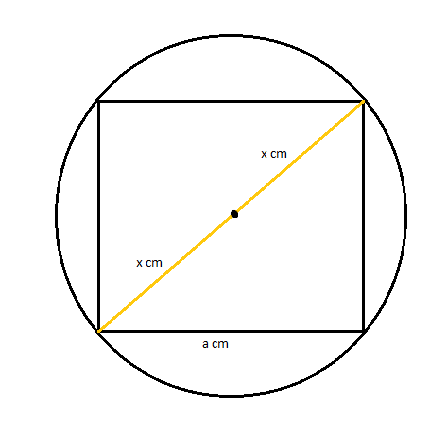QUESTION

# Find the area of a square inscribed in a circle of radius $x{\text{ cm}}$.

Hint: In this question first of all draw the diagram of the square inscribed in the circle then find out the diameter of the circle which will be the diagonal of the square. Now we just need to find the length of the side of the square. Then we just need to use the area formula of squares to get the required answer.

Given radius of the circle $= x{\text{ cm}}$
We know that diameter of the circle is equal to double of the radius of the circle.
Diameter of the circle $d = 2r = 2\left( x \right) = 2x{\text{ cm}}$
We know that the diagonal of a square inscribed in a circle is equal to the diameter of the circle.
So, length of diagonal of the square $D = 2x{\text{ cm}}$
The diagram of the square inscribed in the circle is given as below:We know that if $D$ is the length of the diameter of a square then the length of its side is given by $\dfrac{D}{{\sqrt 2 }}$.
Hence the length of the square inscribed in the circle $a = \dfrac{D}{{\sqrt 2 }} = \dfrac{{2x}}{{\sqrt 2 }} = \sqrt 2 x{\text{ cm}}$
We know the area of the square with side $a{\text{ cm}}$ is given by ${a^2}{\text{ c}}{{\text{m}}^2}$.
Hence the area of the square inscribed in the circle $= {\left( {\sqrt 2 x} \right)^2} = 2{x^2}{\text{ c}}{{\text{m}}^2}$
Thus, the area of the square inscribed in a circle of radius $x{\text{ cm}}$ is $2{x^2}{\text{ c}}{{\text{m}}^2}$.

Note: Diameter of the circle is equal to double of the radius of the circle. Diagonal of a square inscribed in a circle is equal to the diameter of the circle. If $D$ is the length of the diameter of a square then the length of its side is given by $\dfrac{D}{{\sqrt 2 }}$.